# Forms of learning in ai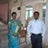Assistant Professor at Vel Tech MultiTech Dr.Rangarajan Dr.Sakunthala Engineering College à Vel Tech MultiTech Dr.Rangarajan Dr.Sakunthala Engineering College
29 Apr 2020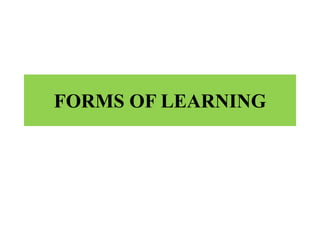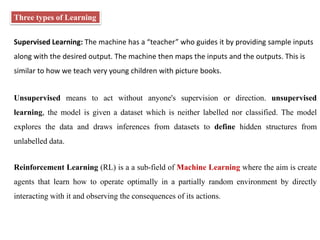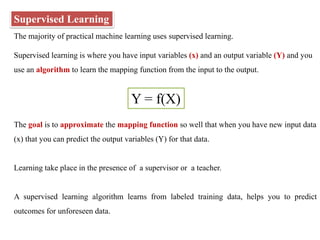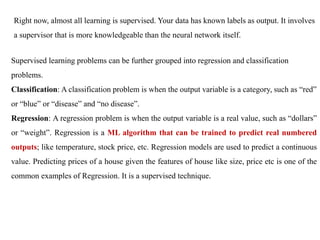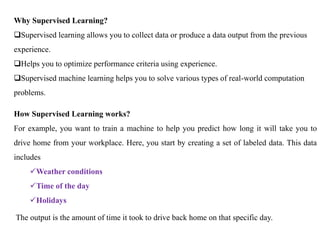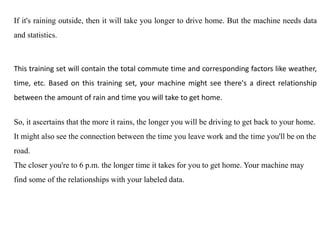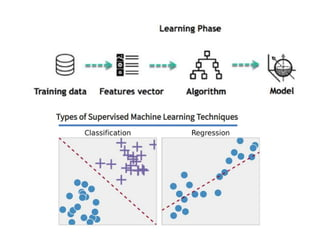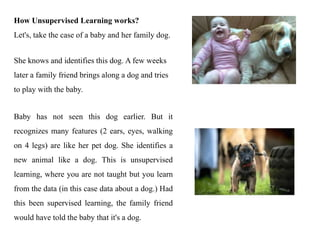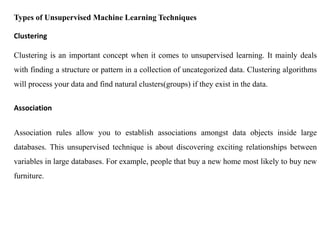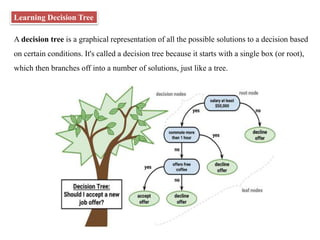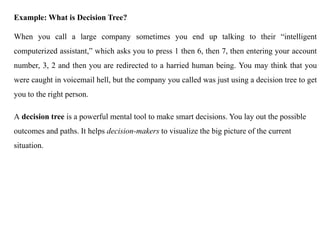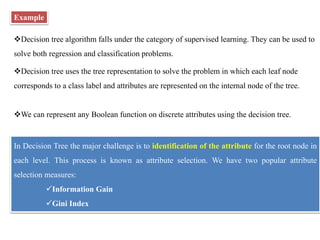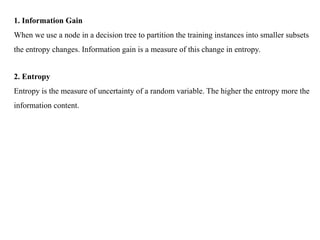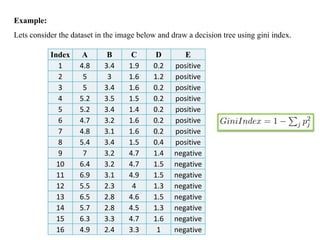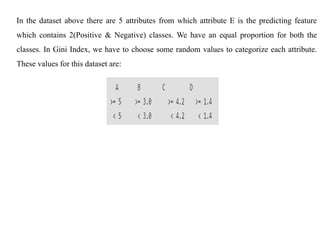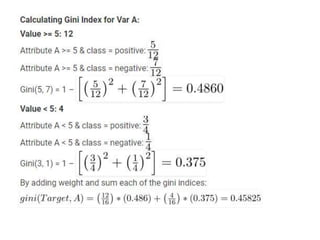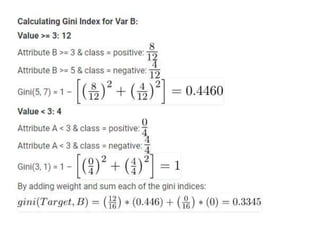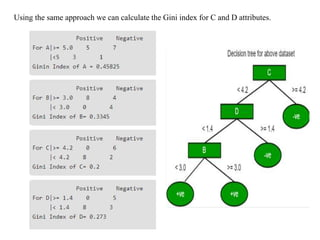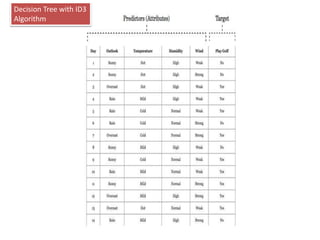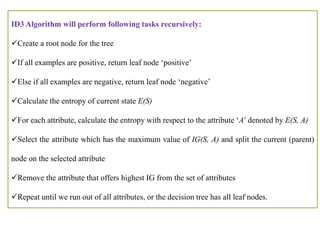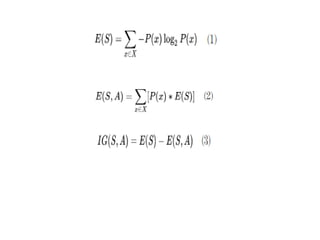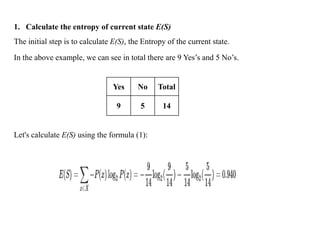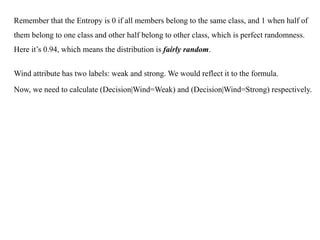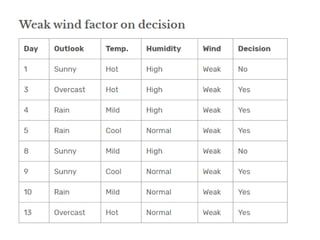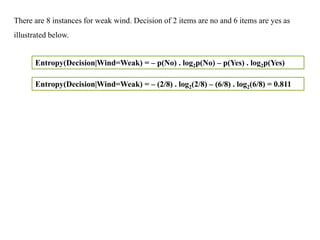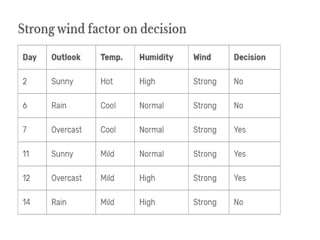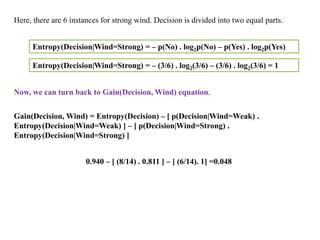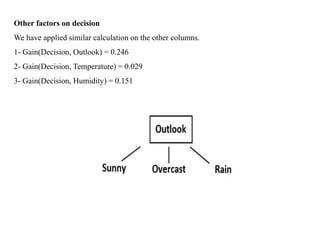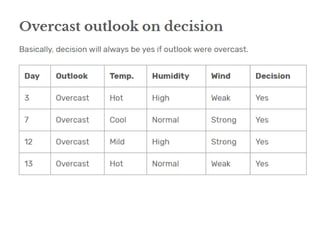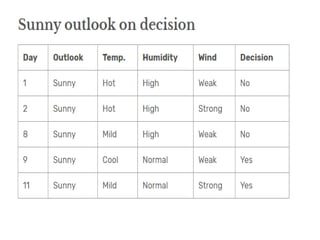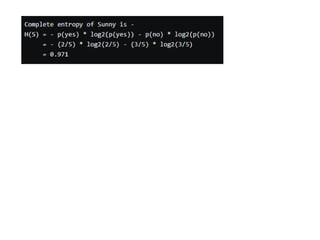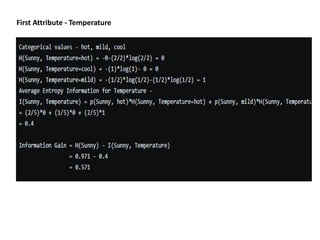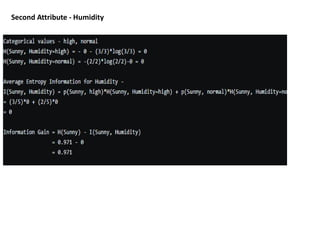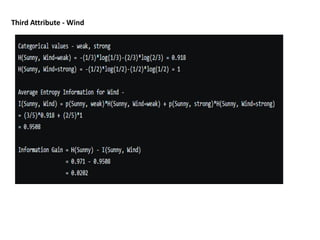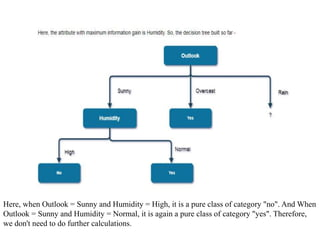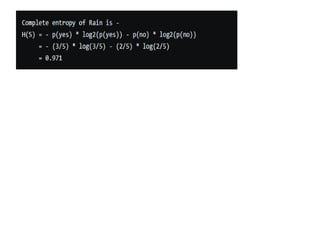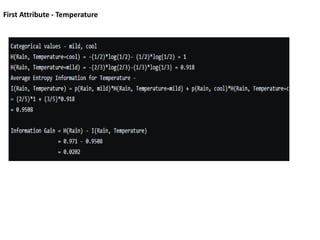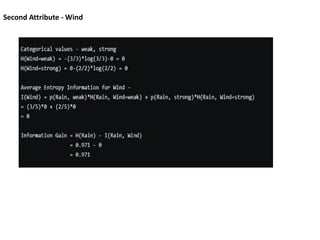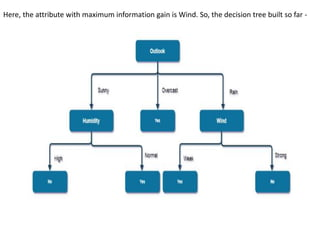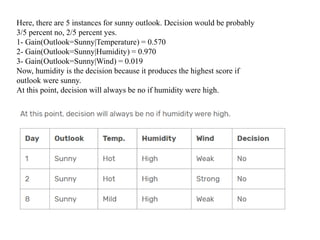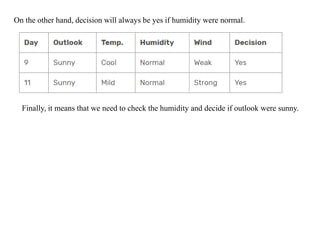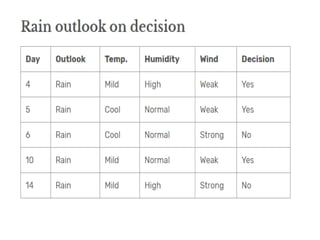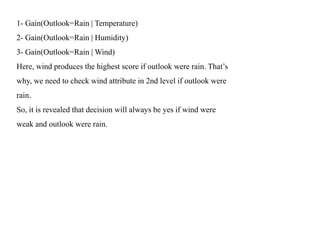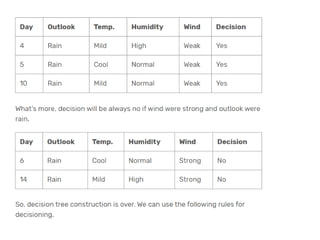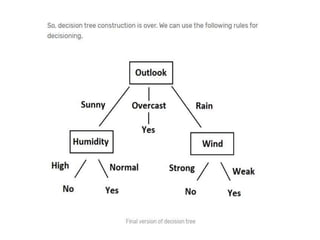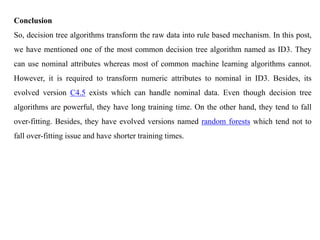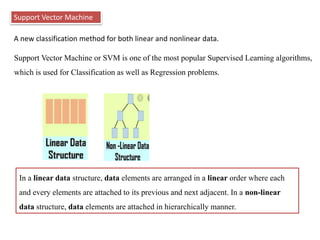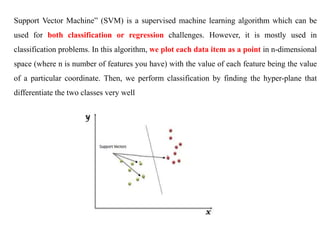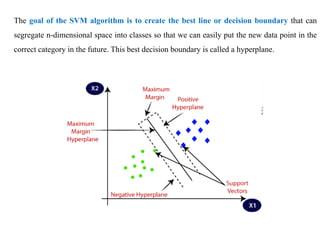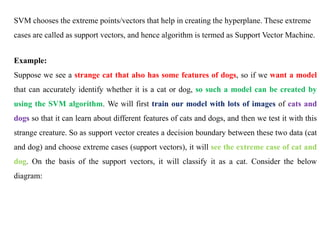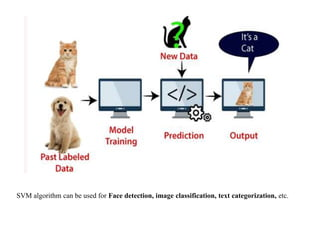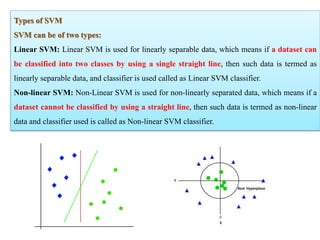1 sur 52

### Forms of learning in ai

• 2. Three types of Learning Supervised Learning: The machine has a “teacher” who guides it by providing sample inputs along with the desired output. The machine then maps the inputs and the outputs. This is similar to how we teach very young children with picture books. Unsupervised means to act without anyone's supervision or direction. unsupervised learning, the model is given a dataset which is neither labelled nor classified. The model explores the data and draws inferences from datasets to define hidden structures from unlabelled data. Reinforcement Learning (RL) is a a sub-field of Machine Learning where the aim is create agents that learn how to operate optimally in a partially random environment by directly interacting with it and observing the consequences of its actions.
• 3. Supervised Learning The majority of practical machine learning uses supervised learning. Supervised learning is where you have input variables (x) and an output variable (Y) and you use an algorithm to learn the mapping function from the input to the output. Y = f(X) The goal is to approximate the mapping function so well that when you have new input data (x) that you can predict the output variables (Y) for that data. Learning take place in the presence of a supervisor or a teacher. A supervised learning algorithm learns from labeled training data, helps you to predict outcomes for unforeseen data.
• 4. Right now, almost all learning is supervised. Your data has known labels as output. It involves a supervisor that is more knowledgeable than the neural network itself. Supervised learning problems can be further grouped into regression and classification problems. Classification: A classification problem is when the output variable is a category, such as “red” or “blue” or “disease” and “no disease”. Regression: A regression problem is when the output variable is a real value, such as “dollars” or “weight”. Regression is a ML algorithm that can be trained to predict real numbered outputs; like temperature, stock price, etc. Regression models are used to predict a continuous value. Predicting prices of a house given the features of house like size, price etc is one of the common examples of Regression. It is a supervised technique.
• 5. Why Supervised Learning? Supervised learning allows you to collect data or produce a data output from the previous experience. Helps you to optimize performance criteria using experience. Supervised machine learning helps you to solve various types of real-world computation problems. How Supervised Learning works? For example, you want to train a machine to help you predict how long it will take you to drive home from your workplace. Here, you start by creating a set of labeled data. This data includes Weather conditions Time of the day Holidays The output is the amount of time it took to drive back home on that specific day.
• 6. If it's raining outside, then it will take you longer to drive home. But the machine needs data and statistics. This training set will contain the total commute time and corresponding factors like weather, time, etc. Based on this training set, your machine might see there's a direct relationship between the amount of rain and time you will take to get home. So, it ascertains that the more it rains, the longer you will be driving to get back to your home. It might also see the connection between the time you leave work and the time you'll be on the road. The closer you're to 6 p.m. the longer time it takes for you to get home. Your machine may find some of the relationships with your labeled data.
• 8. How Unsupervised Learning works? Let's, take the case of a baby and her family dog. She knows and identifies this dog. A few weeks later a family friend brings along a dog and tries to play with the baby. Baby has not seen this dog earlier. But it recognizes many features (2 ears, eyes, walking on 4 legs) are like her pet dog. She identifies a new animal like a dog. This is unsupervised learning, where you are not taught but you learn from the data (in this case data about a dog.) Had this been supervised learning, the family friend would have told the baby that it's a dog.
• 9. Types of Unsupervised Machine Learning Techniques Clustering Clustering is an important concept when it comes to unsupervised learning. It mainly deals with finding a structure or pattern in a collection of uncategorized data. Clustering algorithms will process your data and find natural clusters(groups) if they exist in the data. Association Association rules allow you to establish associations amongst data objects inside large databases. This unsupervised technique is about discovering exciting relationships between variables in large databases. For example, people that buy a new home most likely to buy new furniture.
• 10. Learning Decision Tree A decision tree is a graphical representation of all the possible solutions to a decision based on certain conditions. It's called a decision tree because it starts with a single box (or root), which then branches off into a number of solutions, just like a tree.
• 11. Example: What is Decision Tree? When you call a large company sometimes you end up talking to their “intelligent computerized assistant,” which asks you to press 1 then 6, then 7, then entering your account number, 3, 2 and then you are redirected to a harried human being. You may think that you were caught in voicemail hell, but the company you called was just using a decision tree to get you to the right person. A decision tree is a powerful mental tool to make smart decisions. You lay out the possible outcomes and paths. It helps decision-makers to visualize the big picture of the current situation.
• 12. Example Decision tree algorithm falls under the category of supervised learning. They can be used to solve both regression and classification problems. Decision tree uses the tree representation to solve the problem in which each leaf node corresponds to a class label and attributes are represented on the internal node of the tree. We can represent any Boolean function on discrete attributes using the decision tree. In Decision Tree the major challenge is to identification of the attribute for the root node in each level. This process is known as attribute selection. We have two popular attribute selection measures: Information Gain Gini Index
• 13. 1. Information Gain When we use a node in a decision tree to partition the training instances into smaller subsets the entropy changes. Information gain is a measure of this change in entropy. 2. Entropy Entropy is the measure of uncertainty of a random variable. The higher the entropy more the information content.
• 14. Example: Lets consider the dataset in the image below and draw a decision tree using gini index. Index A B C D E 1 4.8 3.4 1.9 0.2 positive 2 5 3 1.6 1.2 positive 3 5 3.4 1.6 0.2 positive 4 5.2 3.5 1.5 0.2 positive 5 5.2 3.4 1.4 0.2 positive 6 4.7 3.2 1.6 0.2 positive 7 4.8 3.1 1.6 0.2 positive 8 5.4 3.4 1.5 0.4 positive 9 7 3.2 4.7 1.4 negative 10 6.4 3.2 4.7 1.5 negative 11 6.9 3.1 4.9 1.5 negative 12 5.5 2.3 4 1.3 negative 13 6.5 2.8 4.6 1.5 negative 14 5.7 2.8 4.5 1.3 negative 15 6.3 3.3 4.7 1.6 negative 16 4.9 2.4 3.3 1 negative
• 15. In the dataset above there are 5 attributes from which attribute E is the predicting feature which contains 2(Positive & Negative) classes. We have an equal proportion for both the classes. In Gini Index, we have to choose some random values to categorize each attribute. These values for this dataset are:
• 18. Using the same approach we can calculate the Gini index for C and D attributes.
• 19. Decision Tree with ID3 Algorithm
• 20. ID3 Algorithm will perform following tasks recursively: Create a root node for the tree If all examples are positive, return leaf node ‘positive’ Else if all examples are negative, return leaf node ‘negative’ Calculate the entropy of current state E(S) For each attribute, calculate the entropy with respect to the attribute ‘A’ denoted by E(S, A) Select the attribute which has the maximum value of IG(S, A) and split the current (parent) node on the selected attribute Remove the attribute that offers highest IG from the set of attributes Repeat until we run out of all attributes, or the decision tree has all leaf nodes.
• 22. The initial step is to calculate E(S), the Entropy of the current state. 1. Calculate the entropy of current state E(S) In the above example, we can see in total there are 9 Yes’s and 5 No’s. Yes No Total 9 5 14 Let's calculate E(S) using the formula (1):
• 23. Remember that the Entropy is 0 if all members belong to the same class, and 1 when half of them belong to one class and other half belong to other class, which is perfect randomness. Here it’s 0.94, which means the distribution is fairly random. Wind attribute has two labels: weak and strong. We would reflect it to the formula. Now, we need to calculate (Decision|Wind=Weak) and (Decision|Wind=Strong) respectively.
• 25. There are 8 instances for weak wind. Decision of 2 items are no and 6 items are yes as illustrated below. Entropy(Decision|Wind=Weak) = – p(No) . log2p(No) – p(Yes) . log2p(Yes) Entropy(Decision|Wind=Weak) = – (2/8) . log2(2/8) – (6/8) . log2(6/8) = 0.811
• 27. Here, there are 6 instances for strong wind. Decision is divided into two equal parts. Entropy(Decision|Wind=Strong) = – p(No) . log2p(No) – p(Yes) . log2p(Yes) Entropy(Decision|Wind=Strong) = – (3/6) . log2(3/6) – (3/6) . log2(3/6) = 1 Now, we can turn back to Gain(Decision, Wind) equation. Gain(Decision, Wind) = Entropy(Decision) – [ p(Decision|Wind=Weak) . Entropy(Decision|Wind=Weak) ] – [ p(Decision|Wind=Strong) . Entropy(Decision|Wind=Strong) ] 0.940 – [ (8/14) . 0.811 ] – [ (6/14). 1] =0.048
• 28. Other factors on decision We have applied similar calculation on the other columns. 1- Gain(Decision, Outlook) = 0.246 2- Gain(Decision, Temperature) = 0.029 3- Gain(Decision, Humidity) = 0.151
• 32. First Attribute - Temperature
• 33. Second Attribute - Humidity
• 35. Here, when Outlook = Sunny and Humidity = High, it is a pure class of category "no". And When Outlook = Sunny and Humidity = Normal, it is again a pure class of category "yes". Therefore, we don't need to do further calculations.
• 37. First Attribute - Temperature
• 39. Here, the attribute with maximum information gain is Wind. So, the decision tree built so far -
• 40. Here, there are 5 instances for sunny outlook. Decision would be probably 3/5 percent no, 2/5 percent yes. 1- Gain(Outlook=Sunny|Temperature) = 0.570 2- Gain(Outlook=Sunny|Humidity) = 0.970 3- Gain(Outlook=Sunny|Wind) = 0.019 Now, humidity is the decision because it produces the highest score if outlook were sunny. At this point, decision will always be no if humidity were high.
• 41. On the other hand, decision will always be yes if humidity were normal. Finally, it means that we need to check the humidity and decide if outlook were sunny.
• 43. 1- Gain(Outlook=Rain | Temperature) 2- Gain(Outlook=Rain | Humidity) 3- Gain(Outlook=Rain | Wind) Here, wind produces the highest score if outlook were rain. That’s why, we need to check wind attribute in 2nd level if outlook were rain. So, it is revealed that decision will always be yes if wind were weak and outlook were rain.
• 46. Conclusion So, decision tree algorithms transform the raw data into rule based mechanism. In this post, we have mentioned one of the most common decision tree algorithm named as ID3. They can use nominal attributes whereas most of common machine learning algorithms cannot. However, it is required to transform numeric attributes to nominal in ID3. Besides, its evolved version C4.5 exists which can handle nominal data. Even though decision tree algorithms are powerful, they have long training time. On the other hand, they tend to fall over-fitting. Besides, they have evolved versions named random forests which tend not to fall over-fitting issue and have shorter training times.
• 47. Support Vector Machine A new classification method for both linear and nonlinear data. Support Vector Machine or SVM is one of the most popular Supervised Learning algorithms, which is used for Classification as well as Regression problems. In a linear data structure, data elements are arranged in a linear order where each and every elements are attached to its previous and next adjacent. In a non-linear data structure, data elements are attached in hierarchically manner.
• 48. Support Vector Machine” (SVM) is a supervised machine learning algorithm which can be used for both classification or regression challenges. However, it is mostly used in classification problems. In this algorithm, we plot each data item as a point in n-dimensional space (where n is number of features you have) with the value of each feature being the value of a particular coordinate. Then, we perform classification by finding the hyper-plane that differentiate the two classes very well
• 49. The goal of the SVM algorithm is to create the best line or decision boundary that can segregate n-dimensional space into classes so that we can easily put the new data point in the correct category in the future. This best decision boundary is called a hyperplane.
• 50. SVM chooses the extreme points/vectors that help in creating the hyperplane. These extreme cases are called as support vectors, and hence algorithm is termed as Support Vector Machine. Example: Suppose we see a strange cat that also has some features of dogs, so if we want a model that can accurately identify whether it is a cat or dog, so such a model can be created by using the SVM algorithm. We will first train our model with lots of images of cats and dogs so that it can learn about different features of cats and dogs, and then we test it with this strange creature. So as support vector creates a decision boundary between these two data (cat and dog) and choose extreme cases (support vectors), it will see the extreme case of cat and dog. On the basis of the support vectors, it will classify it as a cat. Consider the below diagram:
• 51. SVM algorithm can be used for Face detection, image classification, text categorization, etc.
• 52. Types of SVM SVM can be of two types: Linear SVM: Linear SVM is used for linearly separable data, which means if a dataset can be classified into two classes by using a single straight line, then such data is termed as linearly separable data, and classifier is used called as Linear SVM classifier. Non-linear SVM: Non-Linear SVM is used for non-linearly separated data, which means if a dataset cannot be classified by using a straight line, then such data is termed as non-linear data and classifier used is called as Non-linear SVM classifier.